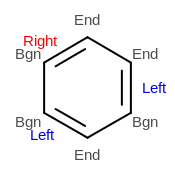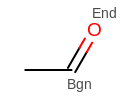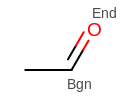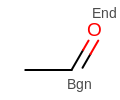# OEBondDisplayPosition¶

This namespace controls how multi-line bonds are rendered. The OEBondDisplayPosition only affects bonds of the following types (as returned by OE2DBondDisplay.GetDisplayType):

The concept of Left and Right are relative to the “begin” and “end” atoms of the associated OEBondBase. The side of Left and Right is defined by standing on the “begin” atom and looking towards the “end” atom.

The following figure shows how both Left and Right double bonds can appear in a benzene ring.Example of various multi-line bond positions

Note

The OE2DBondDisplay does not store pointers to the “begin” and “end” atoms. The “begin” and “end” atoms can be retrieved by accessing the associated OEBondBase through the OEBondDisplayBase.GetBond method and then calling the OEBondBase.GetBgn and OEBondBase.GetEnd methods respectively.

See also

The OEBondDisplayPosition namespace contains the following constants:

Center

Renders a double bond so that the “begin” and “end” atom coordinates end up between each line of the double bond. See example in Figure: Example of a double bond rendered in the center.Example of a double bond rendered in the center

Left

Renders a double bond by drawing a line between the “begin” and “end” atom coordinates, and then drawing a parallel line to the left of that line. See example in Figure: Example of a double bond rendered with its parallel line to the left.Example of a double bond rendered with its parallel line to the left

Right

Renders a double bond by drawing a line between the “begin” and “end” atom coordinates, and then drawing a parallel line to the right of that line. See example in Figure: Example of a double bond rendered with its parallel line to the right.Example of a double bond rendered with its parallel line to the right